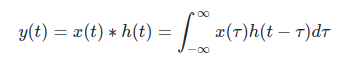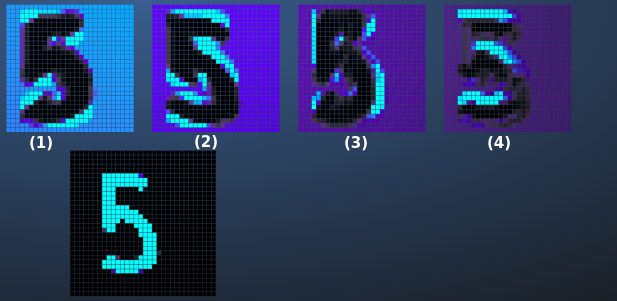The convolutional neural network, from its inception, has been playing a pivotal role in solving real-life problems. For example, in the 1990s, the neural network research group at AT&T developed a convolutional network for reading cheques. Today they are widely used for face recognition, document analysis, and understanding climate change, etc.

Just like humans have eyes that give them the ability to differentiate objects in the real world. Similarly, a convolutional neural network is a type of neural network which allows computers to see things. So, In this blog, we are going to talk about this deep learning technique that revolutionized Artificial Intelligence.

So, why do we call them convolutional neural networks and not the artificial neural network since

CNN also uses the dense or fully-connected layer. Because before a dense layer, it uses several other layers. Those layers sever two purposes:

1. Extracting as many features as possible.
2. Reduce the dimensions of the image data.

One such layer is the convolutional layer that we will be talking about in the latter part of this blog. Because of this layer, we call it a convolutional neural network.

So what is the convolution?

In mathematics, convolution is an operation on two functions that result in a third function expressing how one is influenced by the other. Some applications of this idea are signal processing, reliability, etc.

MathematicallyFig. 1 Mathematical Form.

If you want to know more about the convolutions here, is the link to the video by 3 Blue 1 Brown. For our purposes, we are going to use the idea only, that is, with two signals we are producing a new signal which is influenced by both the signals.

CNN Structure:

So, let's took into the architecture of CNN first. Below is a pictorial representation of the CNN structure. Here, the output of one layer works as input for the next layer.Fig. 2 CNN Structure

1. Convolutional Layer
2. Detector Layer
3. Pooling Layer
4. Fully Connected Layer

1) Convolutional Layer :

It is the first and foremost layer of CNNs. Here the convolution function is applied between image and feature (Filter) so that we get a feature map.

Filter/Kernel - A filter is nothing but a square matrix. It strides over the image and looks for those regions which are similar to that filter.

Fig.3 Represents how convolution operation works. Here, the filter moves over the image, and element-wise matrix multiplication takes place. So, where the filter finds the most similarity, that gets the highest value.Fig. 3 Convolution in Motion.

Fig. 4 represents the maths behind the operation. Although we call it convolution operation but the most accurate terminology is cross-correlation because we are trying to find where the filter has a high or low correlation with the image.Fig. 4 Mathematical Formulae.

Fig 5. represents, by applying more filters' one can create as many features as one want to. The more features we develop, the better will be the model.Fig. 4 Feature Maps.

So how it looks like on the actual image?

So, below we see that image of digit 5 has four different feature maps. Each feature detects something different than the other.Fig.5 Image Representation.

2) Detector Layer :

Some authors consider it as an add-on step to the convolution operation. While many authors, and in our case, it is a separate stage. The detector stage is simply a non-linear function. Images contain many non-linear features like edge, changing pixels, etc. So to preserve this non-linearity of the image, we pass the output of the convolution operation to a non-linear function. ReLu (Rectified Linear Unit) is the most commonly used non-linear function.

3) Pooling layer :

The pooling operation comes after the convolution operation. Here, a square matrix is mapped over the feature to create a summary of statistics of that particular area of the feature. Pooling helps to make representation approximately invariant to the small transitional input or spatial invariance. Even if we give a rotated image to the model, it will be able to detect the image because, by pooling, we are making the model search for that particular feature in the image. Another benefit is that it reduces the dimension of the pixel matrix.

Types of pooling:

a) Max pooling:

In Max pooling, a square matrix with stride, defined by us, is moved over the image. It takes the maximum value of that particular region. Thus giving only dominated elements of the matrix and retaining the feature while reducing the size. Below is the graphical representation of the max pooling. We have applied max-pooling operation on 6*6 matrics resulting in 3*3 matrics. The stride and filter sizes are arbitrary.

b) Average Pooling:

It is similar to the max-pooling, but the only difference here is, instead of taking the maximum, we take the average of that particular region. Below is the graphical representation of the max pooling. We have applied max-pooling operation on 6*6 matrics resulting in 3*3 matrics. The stride and filter sizes are arbitrary.Fig. 7 Average Pooling.

Note - We cannot say that average pooling is better than max pooling or vice-versa. This thing varies from problem to problem. In some cases, Max pooling outperforms the Average pooling. In some cases, Average pooling performs better. Also, there are many other pooling operations like sum pooling, L2 norm, etc.

4) Fully connected layer :

It is a traditional artificial neural network. After applying the pooling operation, we flatten the output from the pooling layer and feed it to the artificial neural network. ANN is the last layer in the CNN structure and with this, we get our outputs. Explaining an artificial neural network is not in the scope of this post. So, I'm assuming that you have a basic understanding of ANNs. Here is the detailed blogpost of the fully connected layer.
Here is the Youtube video in which I have implemented CNN using Tensorflow.
.

Below is the fully developed CNN used for digit classification. Here is the link to the website, this guy has done a great job. This website lets you visualize the change in image at each layer. Go ahead and try yourself.

Note - it's not mandatory to use only one convolutional layer. We can build a more complex network by having multiple convolutional layers. Even the given website uses multiple convolution layers.

Here is the basic python implementation of CNN using Keras. I recommend going through the notebook. It will help you to solidify your understanding of CNN. The dataset is Fashion-MNIST, which is quite popular. It consists of 60,000 training examples and 10,000 test examples. The specialty of this dataset is that it is a  successor of the hand-written MNIST dataset that was used for benchmarking machine learning algorithms. Here is more information about the dataset.